# Diameter

## Table of Contents

 1 Introduction to Circle 2 The Diameter of a Circle 3 Diameter Symbols 4 How do you find a Diameter? 5 Important Notes on Diameter 6 Solved Examples on Diameter 7 Challenging Questions on Diameter 8 Practice Questions on Diameter 9 Maths Olympiad Sample Papers 10 Frequently Asked Questions (FAQs)

We at Cuemath believe that Math is a life skill. Our Math Experts focus on the “Why” behind the “What.” Students can explore from a huge range of interactive worksheets, visuals, simulations, practice tests, and more to understand a concept in depth.

Book a FREE trial class today! and experience Cuemath’s LIVE Online Class with your child.

## Introduction to Circle

A circle is a two-dimensional plane figure. It is a collection of points in which all the points are equidistant from the center point  $$\text {O}$$.

Learn more about the parts of a circle here.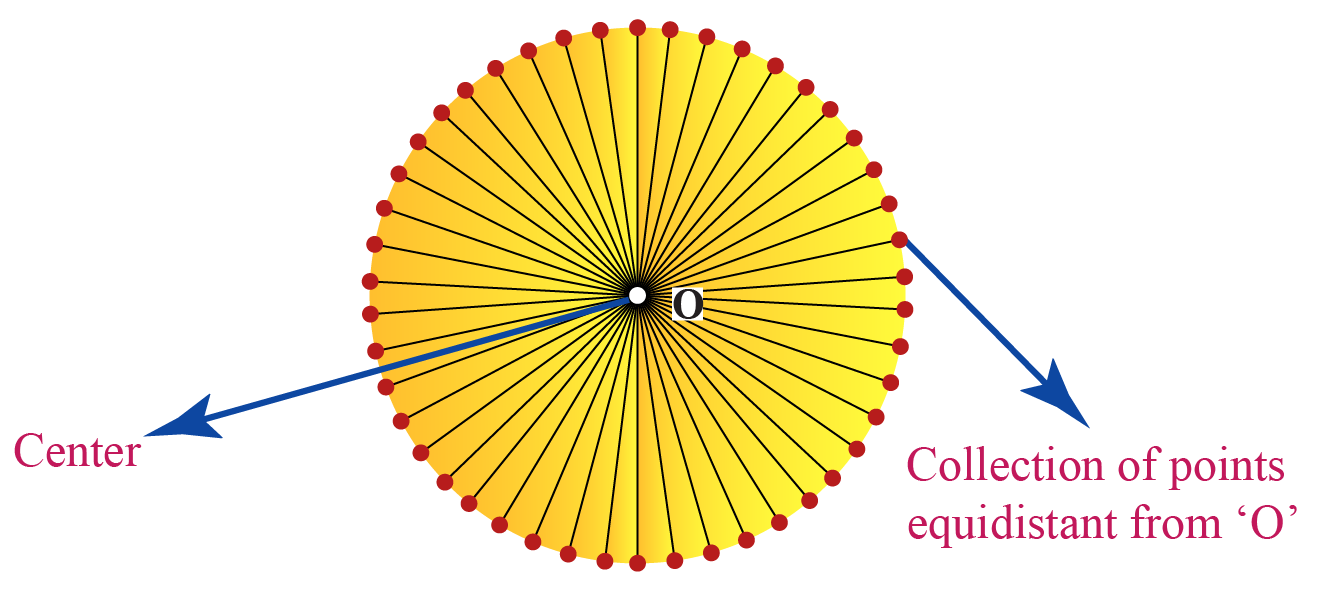Here are some real-life examples of objects which are circular in shape.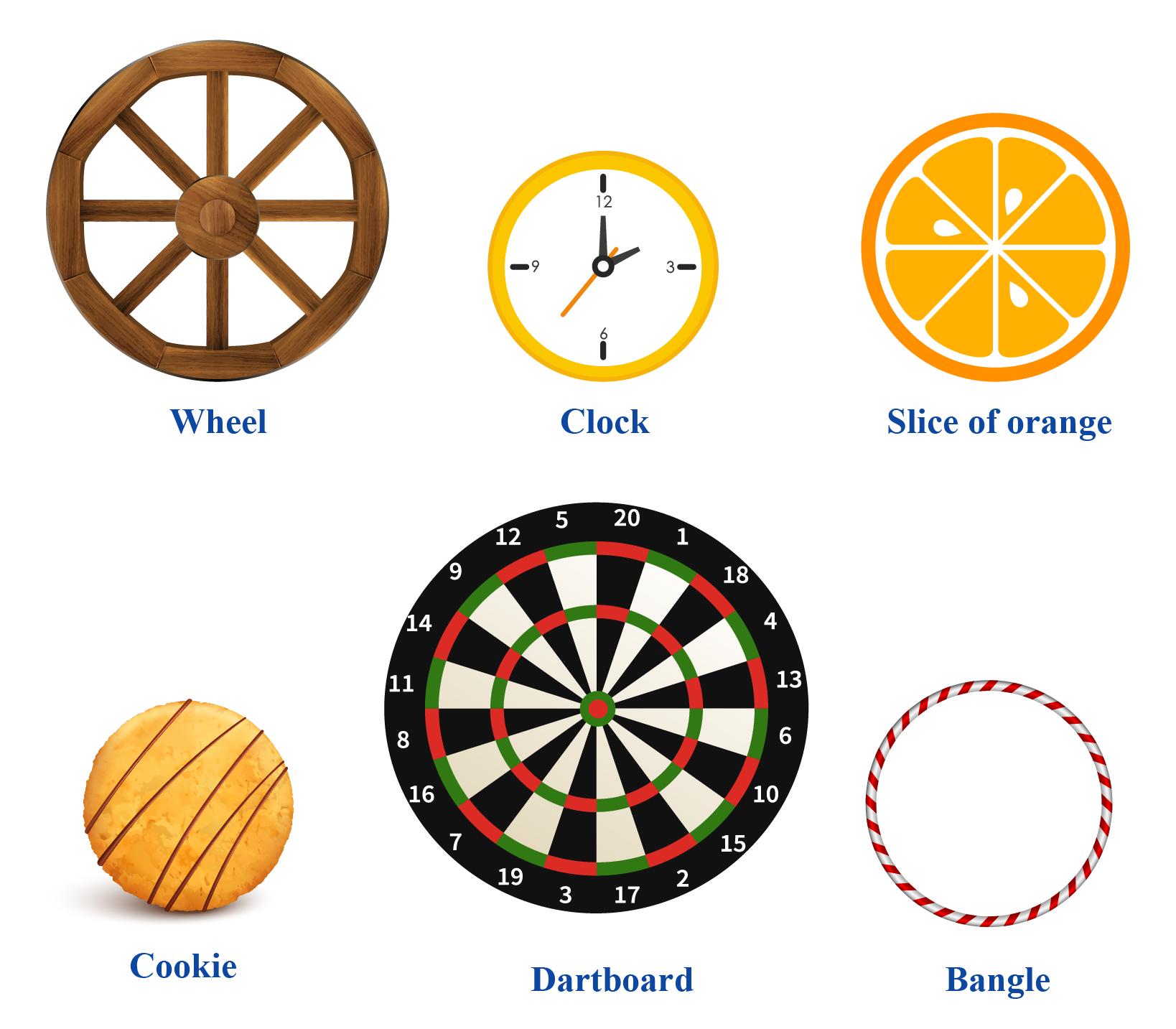Help your child score higher with Cuemath’s proprietary FREE Diagnostic Test. Get access to detailed reports, customised learning plans and a FREE counselling session. Attempt the test now.

## The Diameter of a Circle

The diameter of a circle is a straight line joining a point from one end of the circle to a point on the other end of the circle, passing through the center.

Look at this image.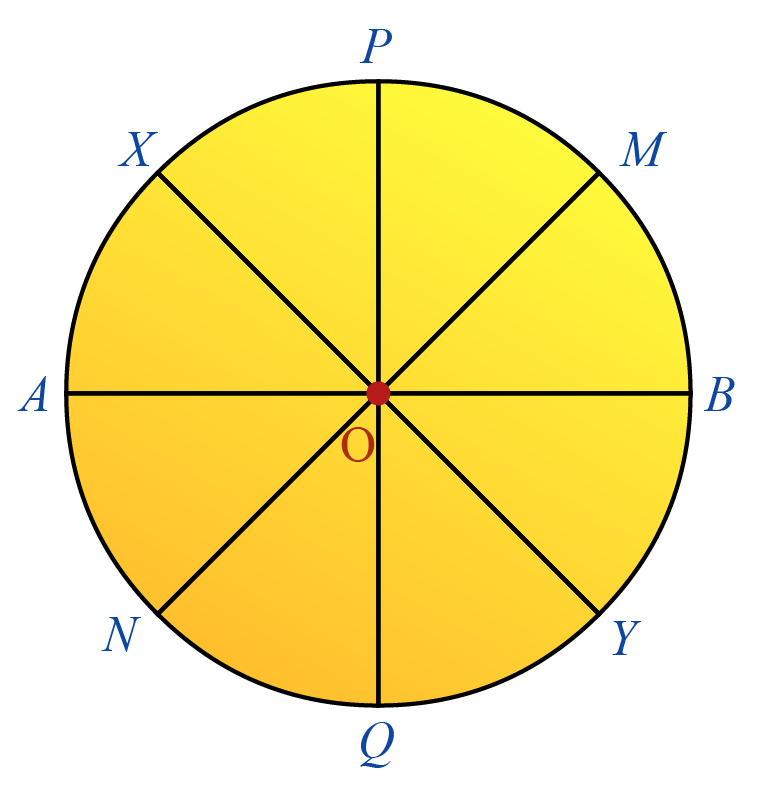Points A, B, M, N, P, Q, X, Y lie on the circle.

'AB' is the line segment which connects the point 'A'  and point 'B'  passing through the center 'O'.

This is the diameter.

Similarly, 'MN', 'PQ', 'XY' also pass through the center  'O'  joining the corresponding points on either side of the circle.

These line segments have the same length.

$$\text{AB = PQ = MN = XY}$$

Note that, 'OA', 'OB', 'OP', 'OQ', 'OM', 'ON', 'OX', 'OY' are half the length of the diameter.

This is called Radius (radii in plural). All radii of the same circle are equal in length.

Radius refers to the line drawn from the center of the circle to an endpoint on the circle.

We can derive the diameter formula from the circumference, the radius, and the area of the circle. Learn more about it here.

## Diameter Symbols

Φ is the symbol for diameter.

The diameter of a circle is abbreviated as  'D', 'DIA', 'diam', 'dia',  'd'.

In lower case, diameter symbol is written as φ or ϕ.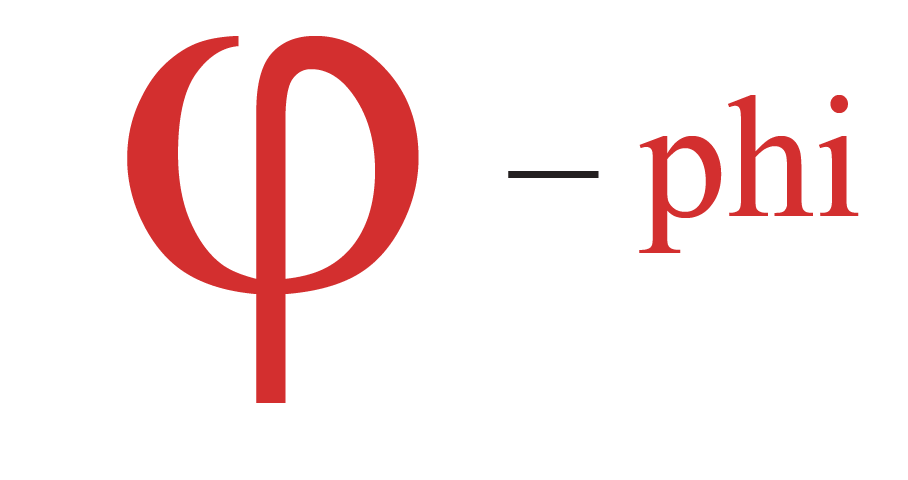The diameter symbol is commonly used in technical specifications and drawings.

A Φ$$25\: \text {mm}$$ means the diameter of the circle is $$25 \: \text {mm}$$.

CLUEless in Math? Check out how CUEMATH Teachers will explain Diameter to your kid using interactive simulations & worksheets so they never have to memorise anything in Math again!

Explore Cuemath Live, Interactive & Personalised Online Classes to make your kid a Math Expert. Book a FREE trial class today!

## How do you find a Diameter?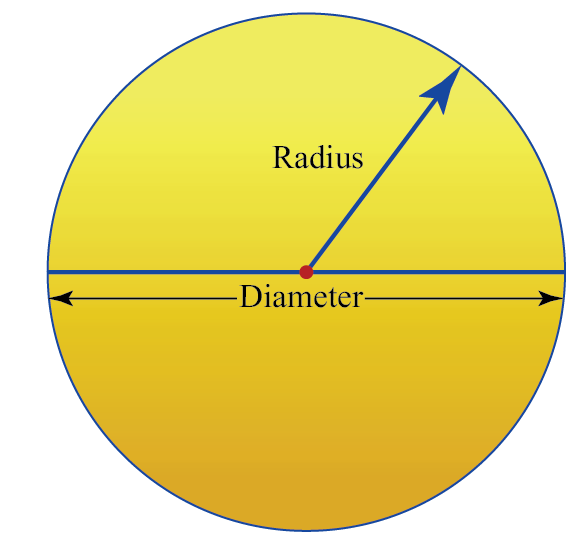Let us understand a few terms before we learn the diameter of a circle formula.

We just learnt that radius ($$\text{r}$$) is the length of the line segment from the center of the circle to an endpoint on the circle.

Circumference ($$\text{C}$$) refers to the enclosed boundary of the circle. We can say that it is the perimeter of the circle.

We can derive the diameter formula from circumference. Let us solve a few example sums here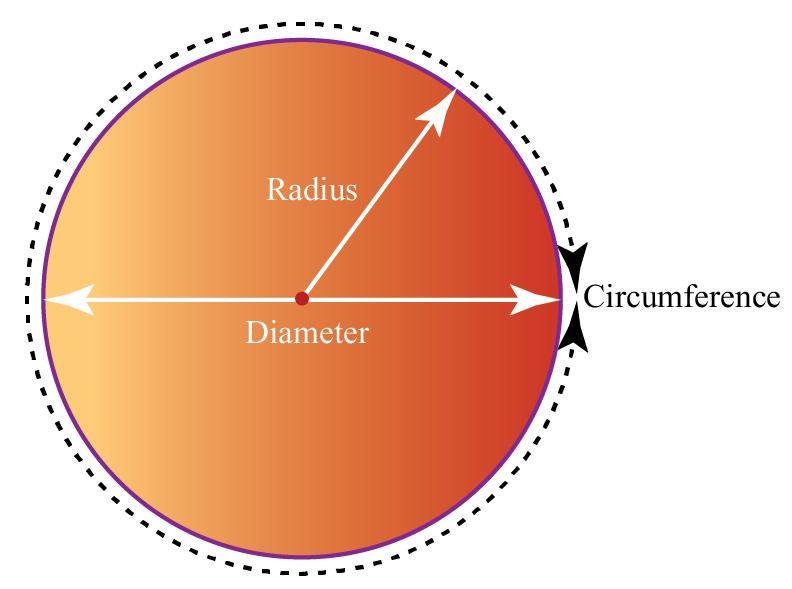$$\text{C= \(\pi$$d}\)

where;

• $$\text C$$ is the circumference
• $$\text d$$ is the diameter
• $$\pi$$ is the constant $$3.14159...$$

The diameter formula when the radius is known is:

 $$\text{Diameter} = 2 \times \text{radius}$$ $$\text {D = 2r}$$

The diameter formula using circumference is:

 $$\text{Diameter} = \frac{\text{Circumference}}{\pi}$$ $\text{D}= \frac{\text{C}}{\pi}$

The diameter formula when the area of the circle is known is :

\begin{align} \text{Area of a circle (A)} &= \pi r^2 \\ \text {Diameter (D)} &= 2\text {r} \end{align}

Simplifying further, we get

 $$D = 2 \sqrt{\frac{ \text {A}}{\pi}}$$

More Important Topics
Numbers
Algebra
Geometry
Measurement
Money
Data
Trigonometry
Calculus